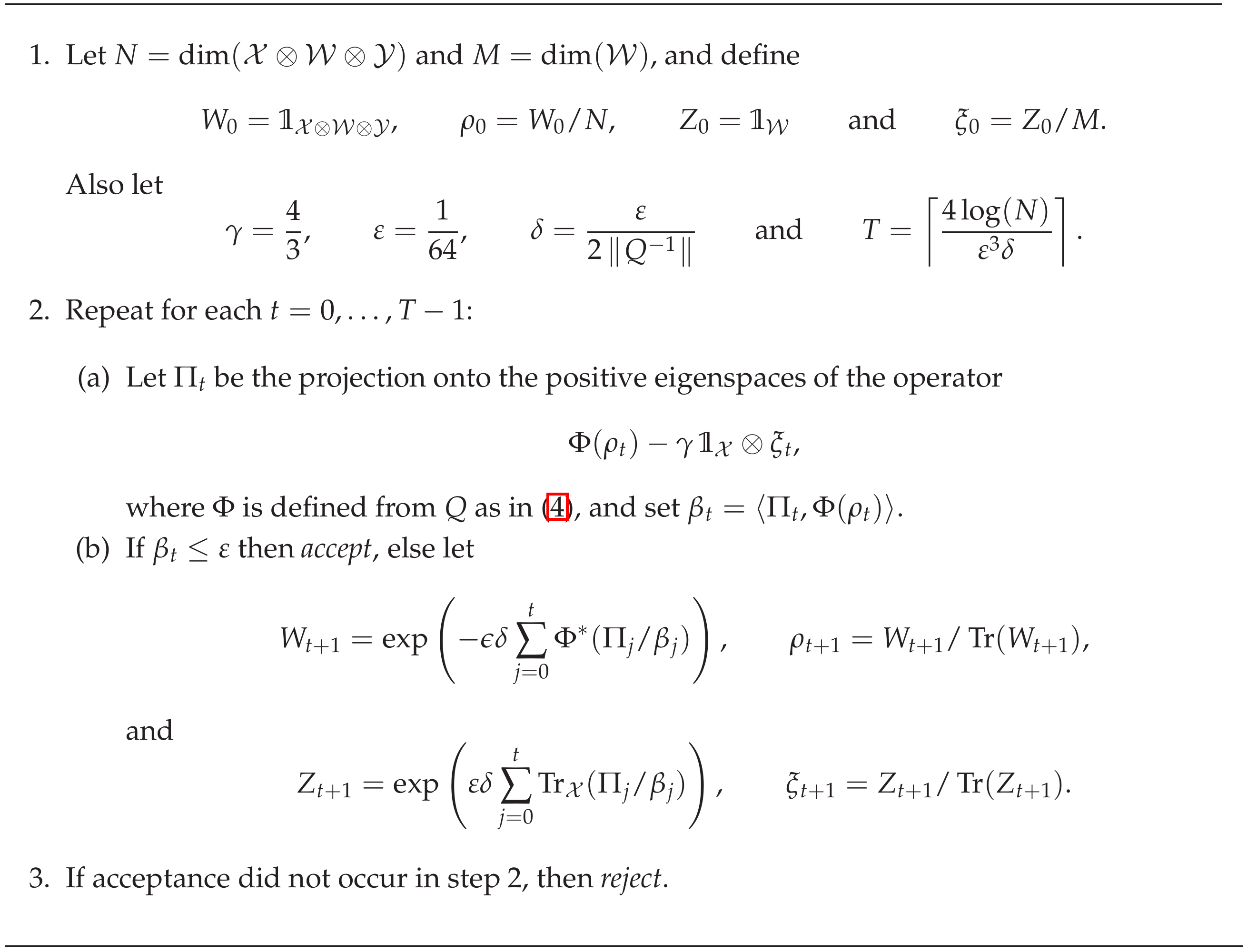STOC Best Papers CollectingThe Annual ACM Symposium on Theory of Computing (STOC), is the flagship conference of SIGACT, the Special Interest Group on Algorithms and Computation Theory, a special interest group of the Association for Computing Machinery (ACM).
Proceedings of the 51st Annual ACM SIGACT Symposium on Theory of Computing, (2019)
The central algorithmic problem for Petri nets is reachability: whether from the given initial configuration there exists a sequence of valid execution steps that reaches the given final configuration0
0
Jakub Tarnawski,
STOC '18: Symposium on Theory of Computing Los Angeles CA USA June, 20..., (2018): 204-213
In Section 5 we show that an -light algorithm for Subtour Partition Cover for an instance I with subtour B can be turned into an approximation algorithm for asymmetric traveling salesman problem with an approximation guarantee of α lb(V \ V(B)) + β + w(B) for any ε > 0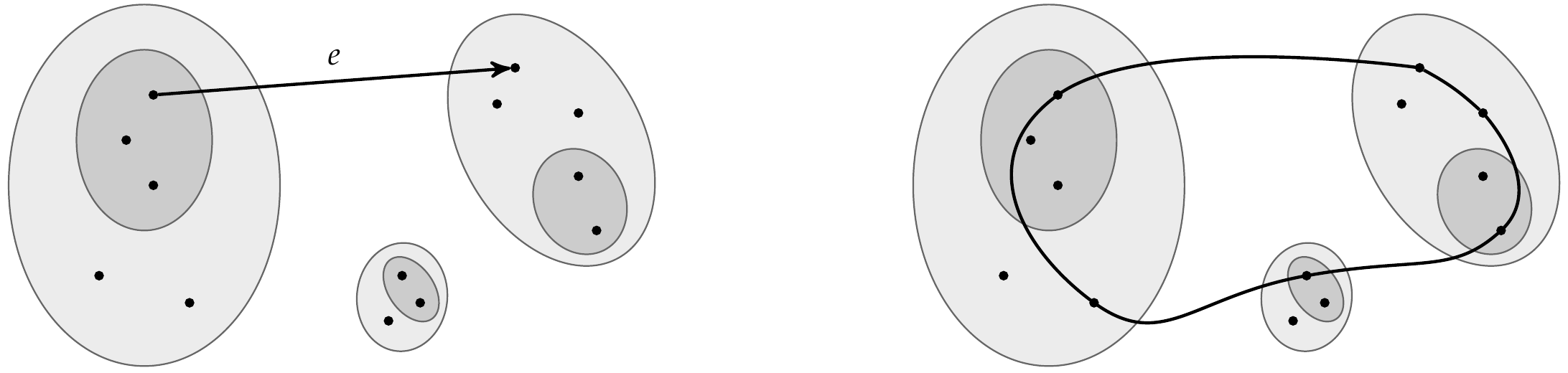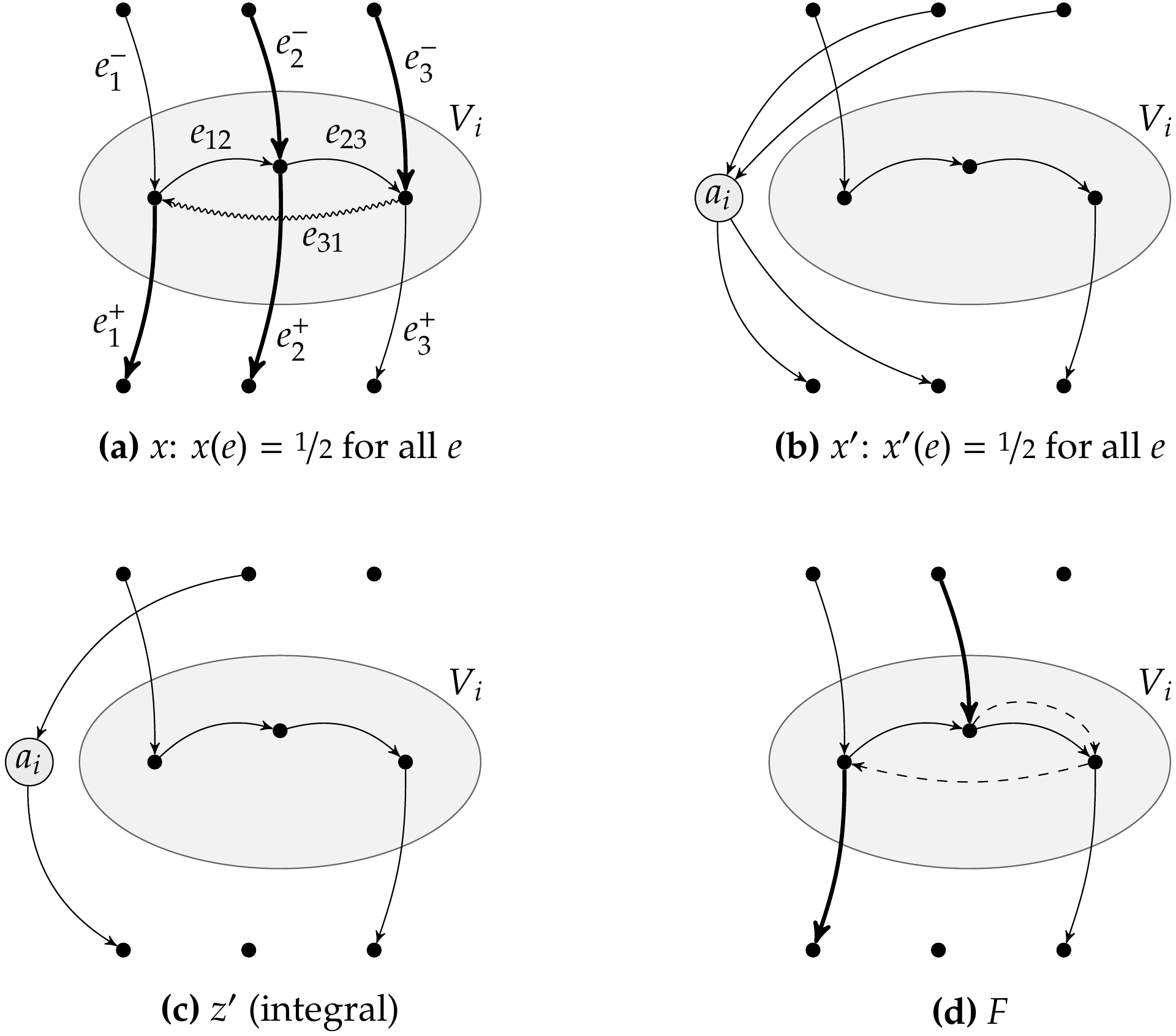0
0
J. ACM, no. 6 (2017): 41:1-41:19
Linear programs are at the heart of combinatorial optimization as they allow to model a large class of polynomial time solvable problems such as flows, matchings and matroids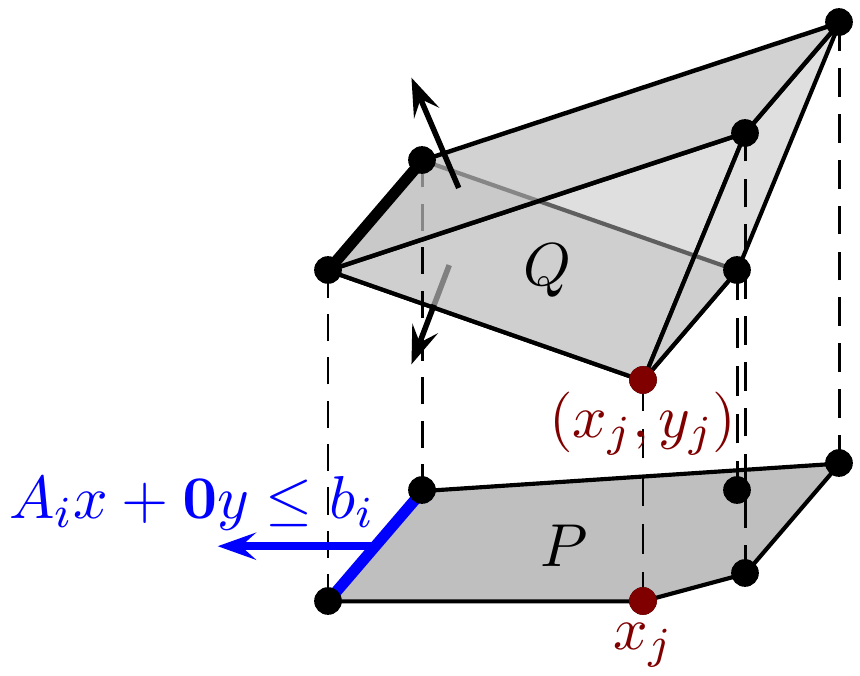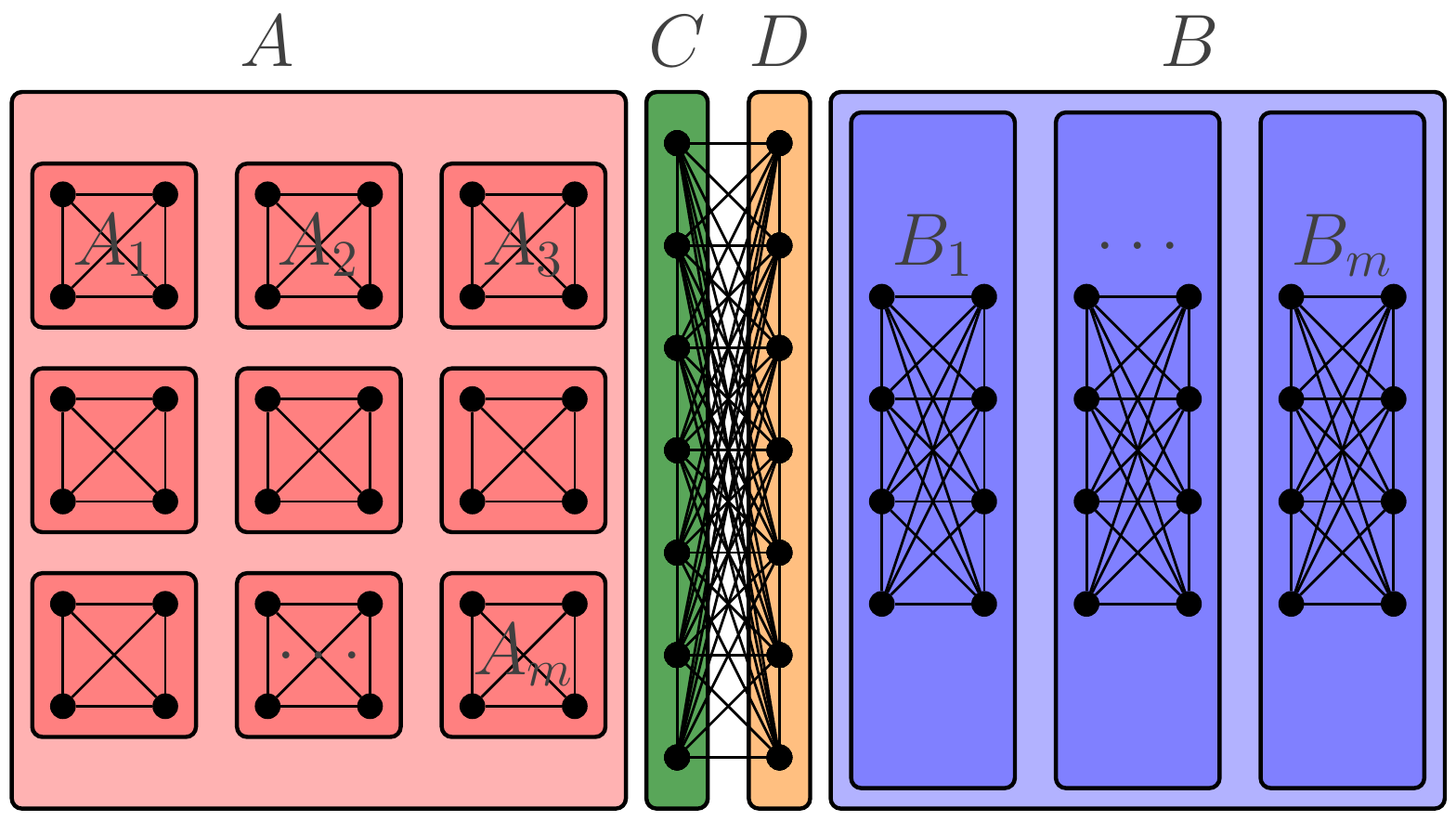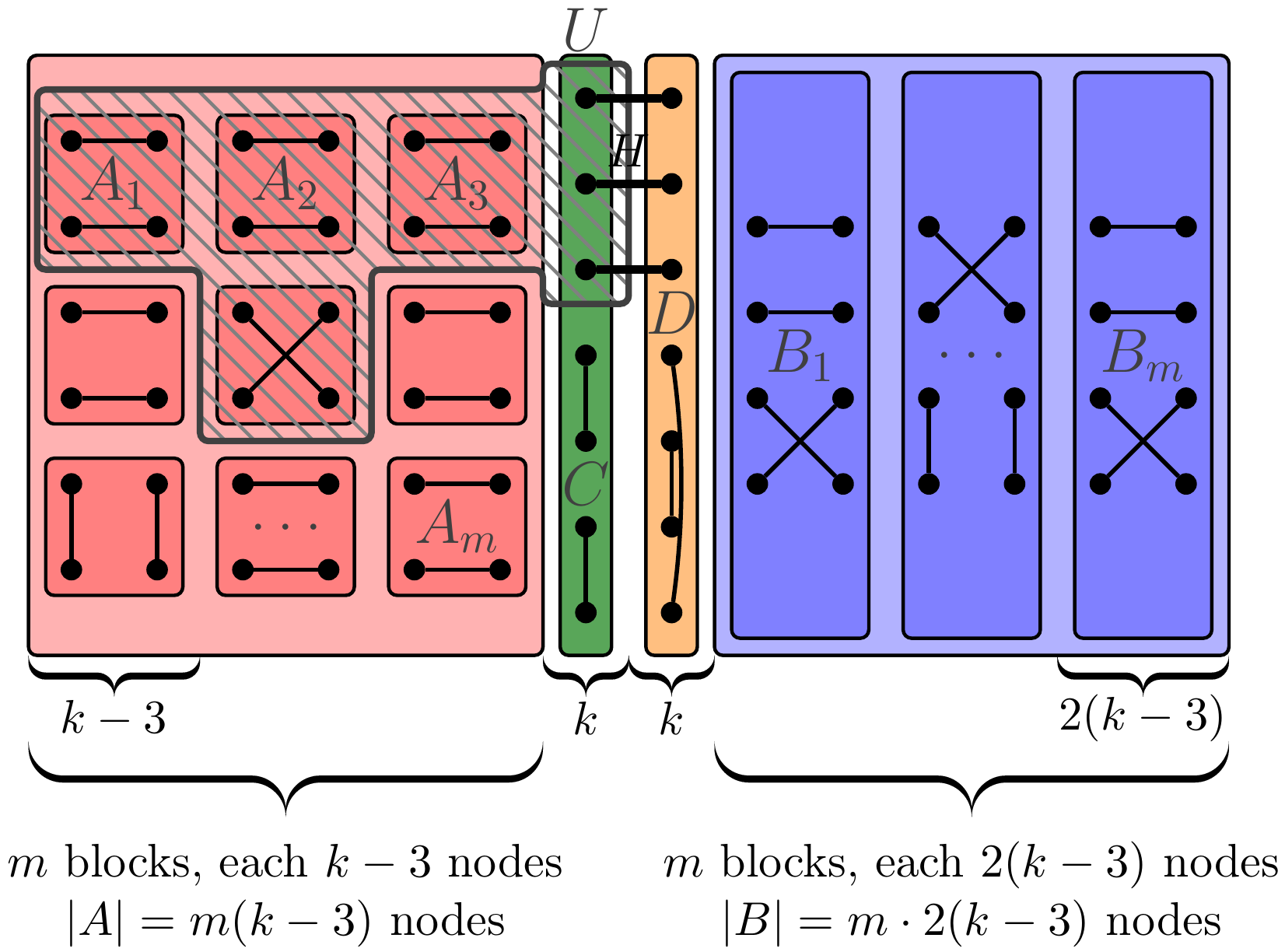0
0
STOC, pp.252-263, (2017)
It is shown that the parity game can be solved in quasipolynomial time. The parameterised parity game â with n nodes and m distinct values (aka colours or priorities) â is proven to be in the class of fixed parameter tractable (FPT) problems when parameterised over m. Both re...0
0
Electronic Colloquium on Computational Complexity (ECCC), (2017)
The question of finding an epsilon-biased set with close to optimal support size, or, equivalently, finding an explicit binary code with distance 1âÑ/2 and rate close to the Gilbert-Varshamov bound, attracted a lot of attention in recent decades. In this paper we solve the pro...0
0
STOC, pp.264-276, (2017)
The algorithms in for the weighted linear matroid parity problem compute the degree of the Pfaffian of another skew-symmetric polynomial matrix, which results in pseudopolynomial complexity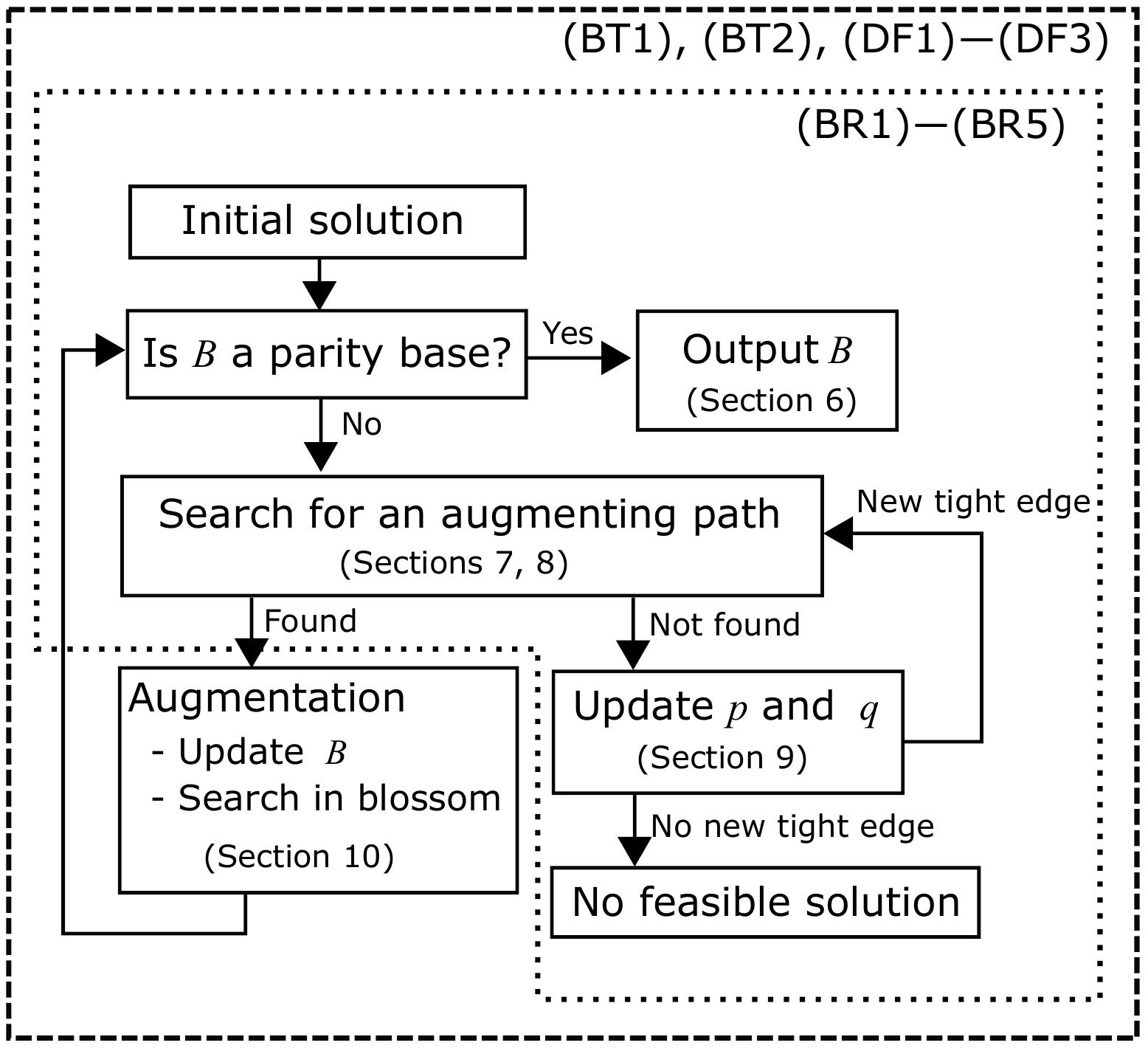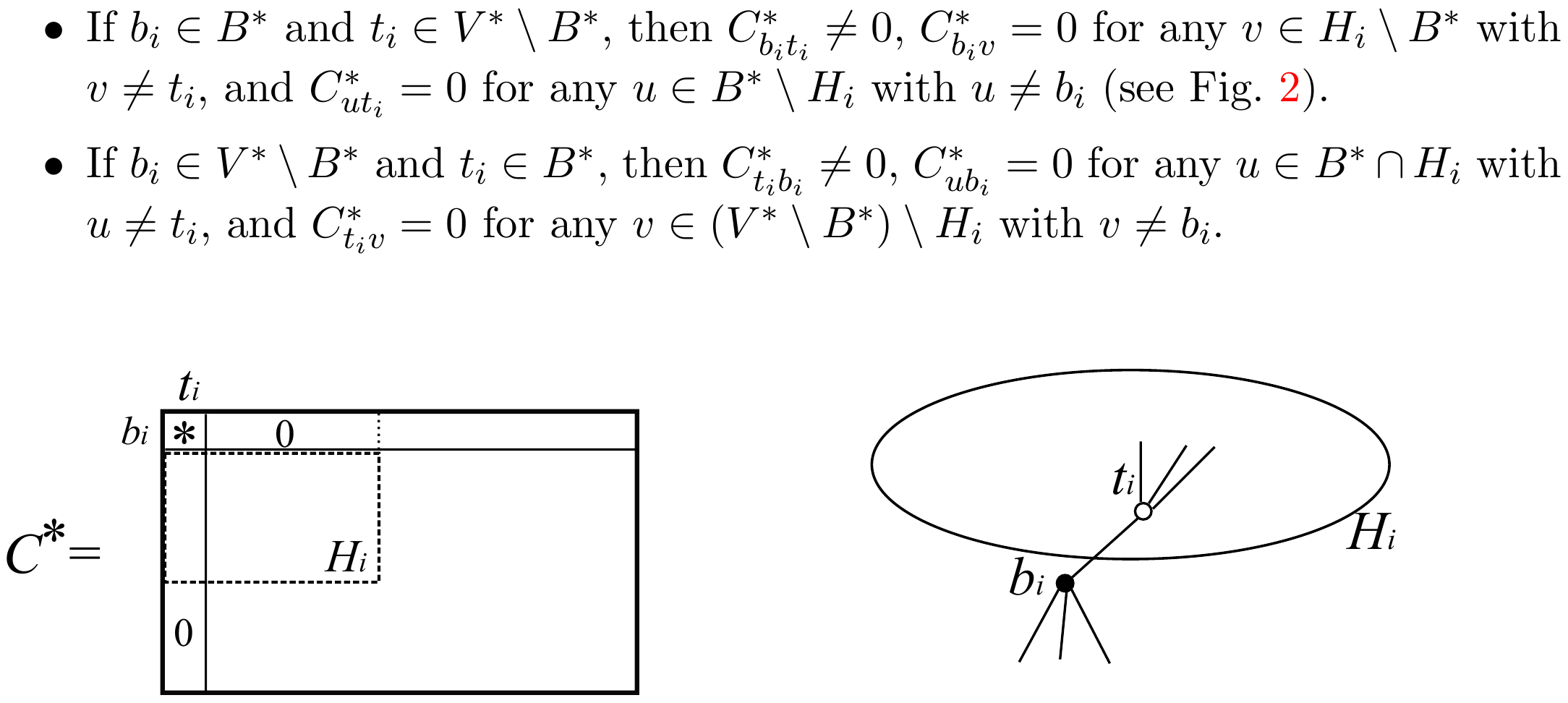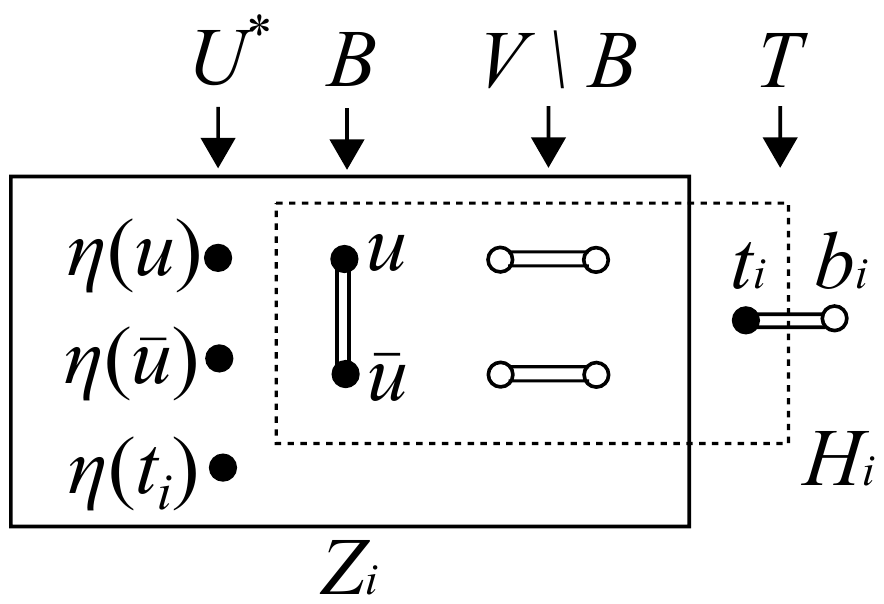0
0
STOC '16: Symposium on Theory of Computing Cambridge MA USA June, 2016, pp.670-683, (2016)
We explicitly construct an extractor for two independent sources on n bits, each with polylogarithmic min-entropy. Our extractor outputs one bit and has polynomially small error. The best previous extractor, by Bourgain, required each source to have min-entropy .499n. A key i...0
0
IEEE Transactions on Information Theory, no. 7 (2016): 4298-4316
Theorem: A sequence of linear codes achieves capacity on a memoryless erasure channel under bit-MAP decoding if its blocklengths are strictly increasing, its code rates converge to some r ∈, and the permutation group1 of each code is doubly transitive0
0
Journal of the ACM (JACM), no. 4 (2016)
One approach to decrease the communication cost is to take m to be a product of r > 2 prime factors in theorem 2.6 to get a larger S-matching vector family where S = {a ∈ Zm: a mod pi ∈ {0, 1} ∀ i ∈ } \ {0} which is of size 2r − 10
0
STOC, pp.684-697, (2016)
We show that the Graph Isomorphism (GI) problem and the more general problems of String Isomorphism (SI) andCoset Intersection (CI) can be solved in quasipolynomial(exp((logn)O(1))) time. The best previous bound for GI was exp(O( √n log n)), where n is the number of vertices (Luk...0
0
symposium on the theory of computing, (2015)
Suppose our goal is to show a lower bound on the size of semidefinite programming relaxations that yield a -approximation on instances of size n0
0
J. ACM, no. 5 (2015): 46:1-46:31
We show an exponential gap between communication complexity and information complexity by giving an explicit example of a partial boolean function with information complexity ≤ O(k), and distributional communication complexity ≥ 2k. This shows that a communication protocol cannot...0
0
Proceedings of the forty-fifth annual ACM symposium on Theory of computing, (2013)
Want to show |SAx|2 = |Ax|2 for all x Can assume columns of A are orthonormal By rotational invariance, SA is a k x d matrix of i.i.d0
0
STOC, pp.457-466, (2013)
We prove that for all integer k ≥ 3, there is a predicate P on k Boolean variables with 2~O(k1/3) accepting assignments that is approximation resistant even on satisfiable instances. That is, given a satisfiable CSP instance with constraint P, we cannot achieve better approximati...0
0
symposium on the theory of computing, (2012): 95-106
The exponential separation between nonnegative rank and PSD rank that we prove here implies more than a super-polynomial lower bound on the extension complexity of the cut polytope0
0
Clinical Orthopaedics and Related Research, (2012): 85-94
With this technique we proved the highest dynamic cell probe lower bound to date under the most natural setting of cell size w = Θ(lg n), namely a lower bound of tq = Ω((lg n/ lg(wtu))2)0
0
Clinical Orthopaedics and Related Research, (2011): 273-282
We introduce a new approach to computing approximately maximum s-t flows and minimum s-t cuts in undirected, capacitated graphs0
0
STOC, pp.283-292, (2011)
The simplex algorithm is among the most widely used algorithms for solving linear programs in practice. With essentially all deterministic pivoting rules it is known, however, to require an exponential number of steps to solve some linear programs. No non-polynomial lower bounds ...0
0
STOC, pp.583-592, (2010)
Our algorithm is based on the following directed-component cut relaxation for the k-restricted Steiner tree problem